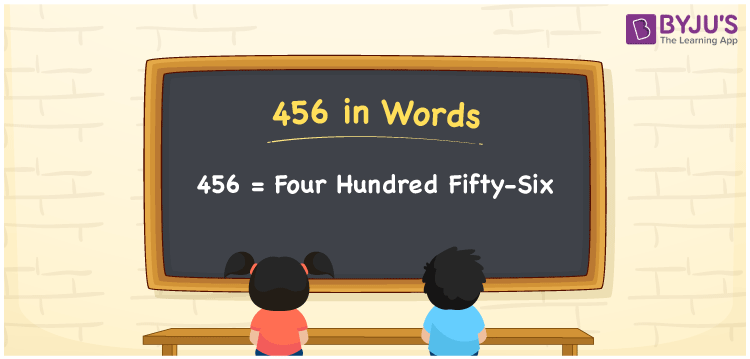# 456 in Words

456 in words can be written as Four Hundred Fifty-Six. The number name of 456 is written taking the place value chart as reference. If you buy coffee powder for Rs. 456, then you can say that “I bought coffee powder for Four Hundred Fifty-Six Rupees”. Numbers in words aims to provide students with complete knowledge of how to write numbers in words using the English alphabet. 456 can be read as “Four Hundred Fifty-Six” in words.

 456 in words Four Hundred Fifty-Six Four Hundred Fifty-Six in Numbers 456

## 456 in English Words## How to Write 456 in Words?

The number 456 has three digits. Students can learn how to prepare the place value chart using the table provided below.

 Hundreds Tens Ones 4 5 6

456 in expanded form can be written as:

4 × Hundred + 5 × Ten + 6 × One

= 4 x 100 + 5 x 10 + 6 x 1

= 400 + 50 + 6

= 456

= Four Hundred Fifty-Six

Hence, 456 in words is written as Four Hundred Fifty-Six.

456 is a natural number that precedes 457 and succeeds 455.

456 in words – Four Hundred Fifty-Six

Is 456 an odd number? – No

Is 456 an even number? – Yes

Is 456 a perfect square number? – No

Is 456 a perfect cube number? – No

Is 456 a prime number? – No

Is 456 a composite number? – Yes

## Frequently Asked Questions on 456 in Words

Q1

### How to write 456 in words?

456 can be written in words as “Four Hundred Fifty-Six”.
Q2

### Write Four Hundred Fifty-Six in numbers.

Four Hundred Fifty-Six in numbers can be written as 456.
Q3

### Is 456 an even number?

456 is an even number as it is completely divisible by 2.